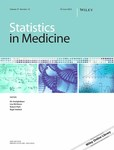# Comments on 'Sample size formula for a win ratio endpoint' by RX Yu and J. GanjuImage credit: John Wiley & Sons, Inc.

### Abstract

We compared two recently published formulas for the sample size calculation based on the win ratio and the win odds tests. Both are general formulas that can incorporate unbalanced randomization and ties. In the case of no ties and both formulas give similar results. The main difference between these formulas is how the alternative hypothesis is formulated (in terms of a win odds or a win ratio). We hope by comparing two recently published formulas on the win ratio/win odds tests and indicating their advantages and disadvantages we will give the statistical and clinical community a better understanding of both formulas, so as to be able to correctly apply them in their research.

Type##### Samvel B. Gasparyan
###### Biostatistician

Biostatistician in cardiovascular trials.